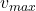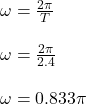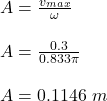An air track glider is attached to a spring. The glider is pulled to the right and released from rest at t=0 seconds. It then oscillates wit

Question

An air track glider is attached to a spring. The glider is pulled to the right and released from rest at t=0 seconds. It then oscillates with a period of 2.40s and a maximum speed of 30.0 cm/s. What is the glider’s position at t=0.300s?

in progress 0
5 months 2021-08-04T07:14:35+00:00 1 Answers 15 views 0

The glider’s position at t=0.300s is 8.1 cm

Explanation:

Given;

maximum speed of the glider,= 30.0 cm/s = 0.3 m/s

period of the oscillation, T = 2.4 s

Maximum speed of oscillation is given by;= ωA

Where;

A is the amplitude of the oscillation

ω is the angular speedThe amplitude of the oscillation is given by;The position of the particle is given by;

x(t) = A cosωt

x(0.3) = (0.1146)cos(0.833π x 0.3)

x(0.3) = (0.1146)cos(0.833π x 0.3)

x(0.3) = (0.1146)cos(45)

x(0.3) = 0.081 m

x(0.3) = 8.1 cm

Therefore, the glider’s position at t=0.300s is 8.1 cm